Coming to Statistica
in 2022/2023

Zoom tutorials
using Explaineverything app
We a record sessions for you to listen to later

CALL STATISTICA NOW

.

E-mail     anne@statistica.com.au

# Place         Willetton, Western Australia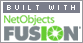# Mathematics & Statistic Tutor Perth - SPSS Help

The  CAST diagram is the wave diagram drawn around a spot rather than along a line. Each segment is one of the quadrants and the sign of the trigonometric function changes depending in which quadrant it lies. From the diagrams below, you will see that between 0 and 90, 360 and 450 etc all the trigonometric functions are positive.  Since the number we have is + 1/2 then we only look at the quadrants which Sine is positive and  simply calculate the angle as shown. You will go 3 times and as you do the angles change on the outside.  Beware when the angle is negative and check which quadrants you need, if the number had been - 1/2 then the two angles needed would be in the two bottom areas from 180 to 270 and 270 to 360.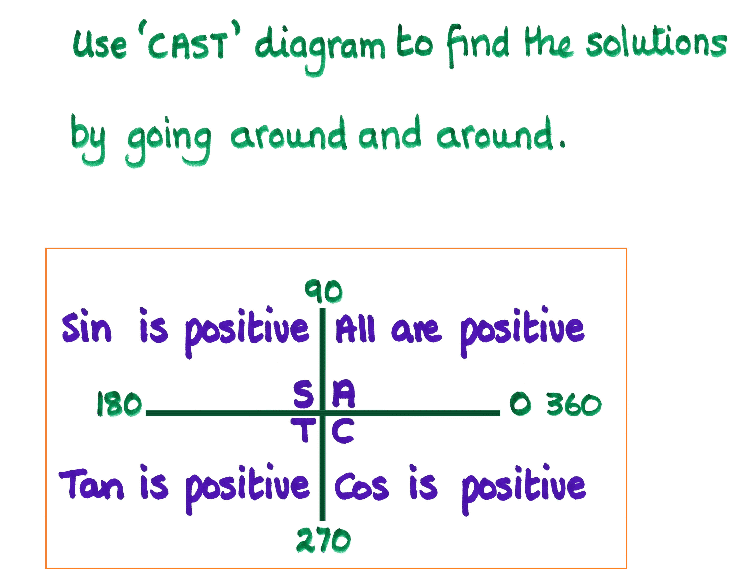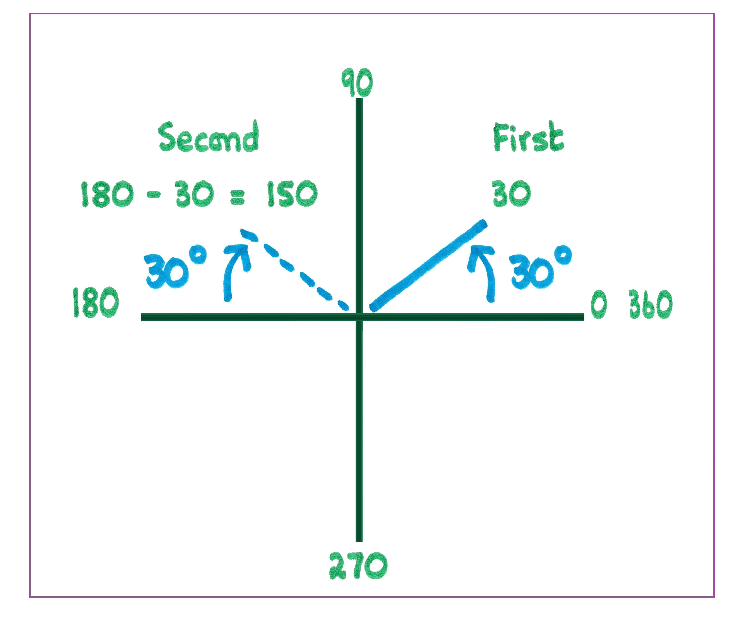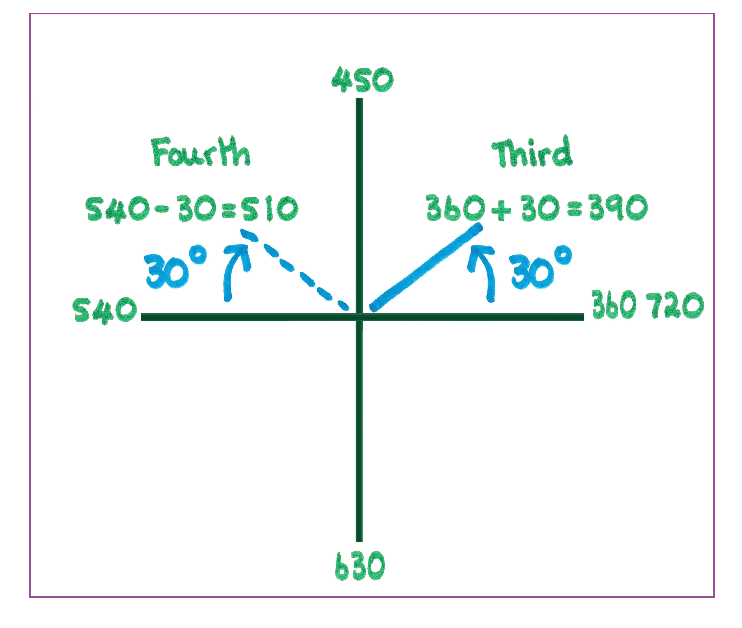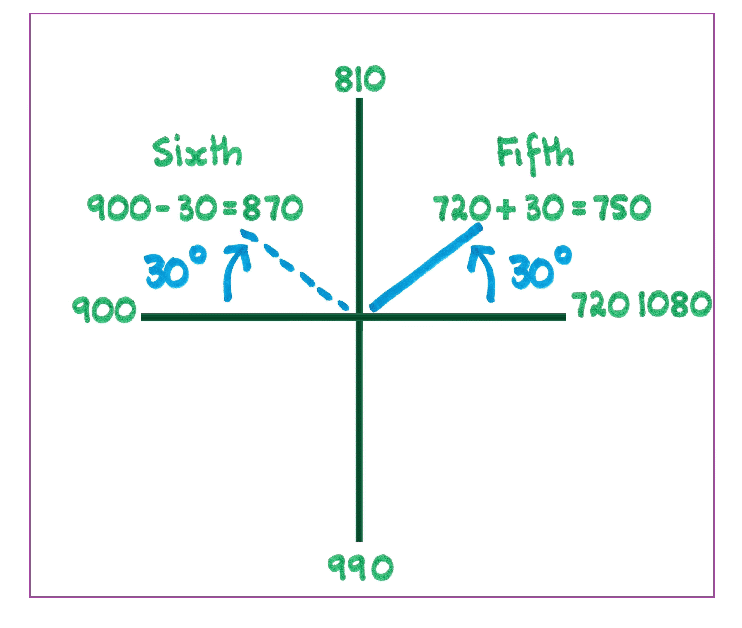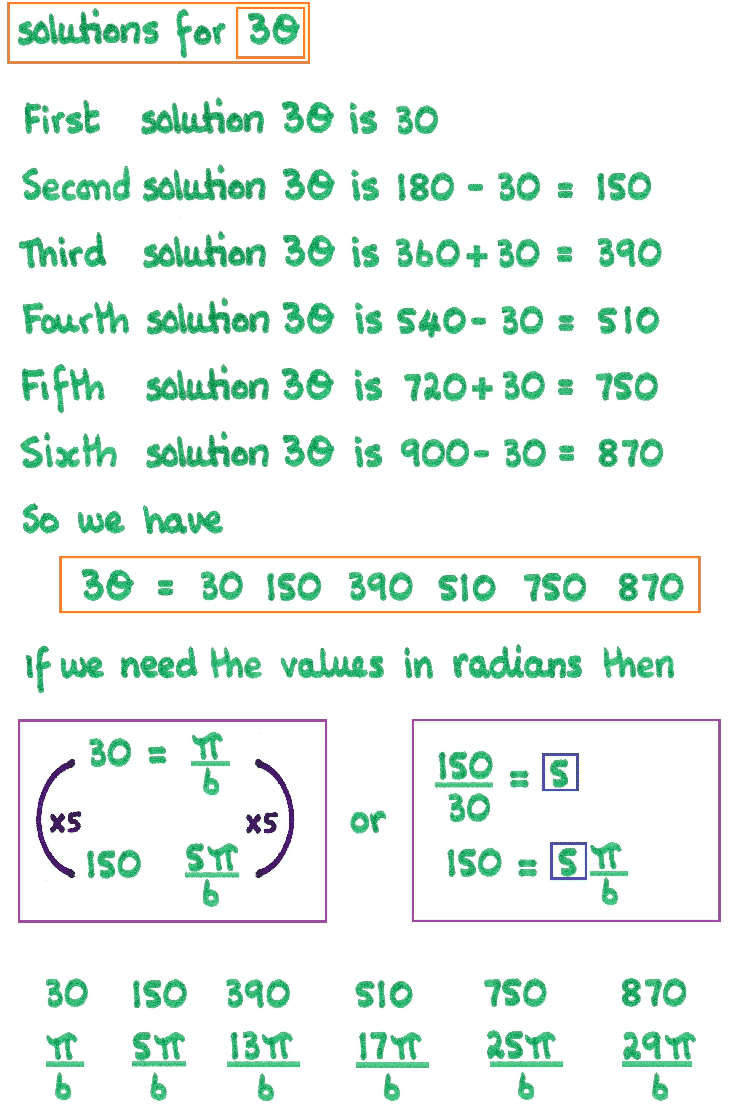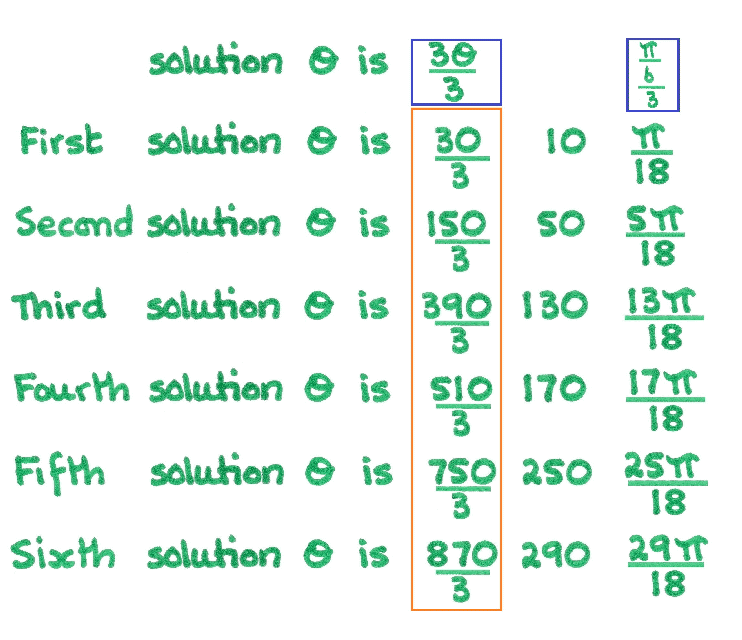## Step Eight[Home] [Parents] [Contact us] [Services] [Mathematics] [Statistics] [School]